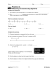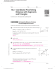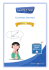Coordinate Geometry

Transcription

Coordinate Geometry
Intro to Applied and PreCalc
Unit: Coordinate Geometry
Distance Homework
√(
)
(
)
1. Find the distance between the following pairs of points to two
y decimal places.
10
Example Solution:
8
C
Distance between points A and B
A
6
Label points
A(2, 6) and B(6, -1)
(
)
(
)
4
2
Substitute into formula:
)
(
)
√(
d
-10
-8
-6
2
-2
2
-2
D
(6  2)  (1 6)
2
-4
-4
(4)2  (7)2
d  16  49
d  65
d
-6
-8
-10
d ≈ 8.06
a) B
and C
b) C
and D
c)
A
and D
d) B
and D
e)
A
and C
Page 1
4
6
B
8
10
x
Intro to Applied and PreCalc
Unit: Coordinate Geometry
2. Bob and Christine want to get together (see map below). Each block is 120 m
by 120 m. Assuming the roads are of negligible width, how far does Bob have
to travel to reach Christine if:
a) he is restricted to the roads?
B
C
b) he can follow a direct path?
y
3. Three vertices of a rectangle ABCD
are given:
A(-3, 1)
5
4
3
B(2, 1)C(-3, 5)
2
1
a) Determine the coordinates of vertex D.
-5
-4
-3
-2
-1
1
-1
2
3
4
x
5
-2
b) Find the lengths of the sides.
-3
-4
-5
c) Determine the length of the diagonal.
4. A triangle has vertices of A(-2, 5), B(5, 3), and C(0, 2). Find the perimeter of
triangle ABC.
y
5
4
3
2
1
Classify the triangle as scalene (no equal
sides), isosceles (two equal sides), or
equilateral (three equal sides).
-5
-4
-3
-2
-1
-1
-2
-3
-4
-5
Page 2
1
2
3
4
5
x
Intro to Applied and PreCalc
Unit: Coordinate Geometry
y
5. P is the point (4, 2). Q is a point on the
y-axis so that PQ = 5. Find the possible
5
4
3
2
1
-5
-4
-3
-2
-1
1
-1
2
3
4
x
5
-2
-3
-4
-5
y
6. Given the following graph of a line,
which of the points (0, 5), (3, 4),
(2, 1), (1, 3), (5, -5) and (-1, 7) do
not lie on the line?
7
6
5
4
3
2
1
-7 -6 -5 -4 -3 -2 -1-1
-2
-3
-4
-5
-6
-7
Distance Homework (Page 8-10)
1.
a) d = 13.45 units
b) d = 11.05 units
d) d = 11.18 units
e) d = 6.32 units
2. a) 360 metres
b) 268.3 metres
3. a) (2, 5)
b) 4 units & 5 units
4.dAB = 7.28
dBC = 5.10
dAC = 3.61
5. (0, 5) & (0, -1)
6. (3, 4) does not lie on the line
Page 3
1
c) d = 11.40 units
c) diagonals = 6.4 units
Perimeter = 15.99 units Scalene triangle
2
3
4
5
6
7
x
Intro to Applied and PreCalc
Unit: Coordinate Geometry
Midpoint Homework
(
(
)
(
)
)
1. Find the midpoint of the following pairs of points.
y
Example:
A
and B
10
8
C
A
(2, 6) and (6, -1)
(
(
) (
)
4
)
 2  6 6  ( 1) 
,


2 
 2
8 5
 , 
2 2
 5
 4, 
or (4, 2.5)
 2
a) B
and C
b) C
and D
6
2
-10
-8
-6
D
-4
-2
2
-2
4
6
8
10
x
B
-4
-6
-8
-10
c) A
and D
2. The midpoint of AB is the origin. B has coordinates of (3, 7). What are the coordinates
of A?
Page 4
Intro to Applied and PreCalc
Unit: Coordinate Geometry
3. One endpoint of a segment AB is A(-3, 6). If the coordinates of the midpoint are (1, 2),
find the coordinates of B.
4. The endpoints of the diameter of a circle are (-6, -2) and (2, 4). Find the circumference
and area of the circle correct to three decimal places.
C   d,
A  r 2

5. A(1, 1), B(7, 3), C(8, 6) and D(2, 4) are the vertices of parallelogram ABCD. Find the
midpoint of AC and the midpoint of BD. Comment on your result.
Midpoint Homework
 7

 2
 9 5
, 
 2 2
1.
a)  1,
2.
A(-3, -7)
3.
(5, -2)
4.
5.
MAC = MBD = 
Page 5
b)  
 3 3
, 
 2 2
c)  
C = 31.416 units
9 7
,  Midpoints are the same.
2 2
A = 78.540 u2
Intro to Applied and PreCalc
Unit: Coordinate Geometry
Slope Homework: Textbook Page 132 #1-4, 6, 13 (Answers on Page 276)
Table of Values Graphing Homework:
Page 6

Page 1 Proving a triangle is a right triangle Method 1: Show two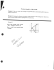Algebra with shapes How to . . .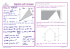HSM12CC_GM_06_09_CM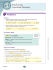5.1 Midsegment Theorem and Coordinate Proof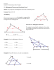Solve the problems below to answer the riddle: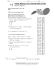The Midpoint Formula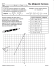Reteach Sec 1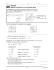STUDY GUIDE for Unit 1 Test Geometry 1. Z?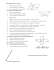Geometry Worksheet Name: Hodge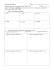1-2 Practice A